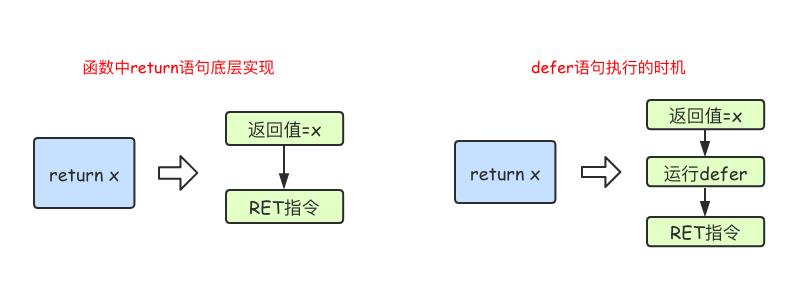Go 函数
2020.09.08 16:10 2020.09.08 16:10

### 函数

Go语言中支持函数、匿名函数和闭包，并且函数在Go语言中属于“一等公民”。

#### 定义

Go语言中定义函数使用`func`关键字，具体格式如下：

``````func 函数名(参数)(返回值){
函数体
}``````

• 函数名：由字母、数字、下划线组成。但函数名的第一个字母不能是数字。在同一个包内，函数名也称不能重名（包的概念详见后文）。
• 参数：参数由参数变量和参数变量的类型组成，多个参数之间使用`,`分隔。
• 返回值：返回值由返回值变量和其变量类型组成，也可以只写返回值的类型，多个返回值必须用`()`包裹，并用`,`分隔。
• 函数体：实现指定功能的代码块。

``````func sum(a int, b int) int {
return a + b
}``````

``````func main() {
fmt.Println(sum(1, 3))
}``````

#### 简写

``````func intSum(x, y int) int {
return x + y
}``````

``````func intSum2(x ...int) int {
fmt.Println(x) //x是一个切片
sum := 0
for _, v := range x {
sum = sum + v
}
return sum
}``````

``````ret1 := intSum2()
ret2 := intSum2(10)
ret3 := intSum2(10, 20)
ret4 := intSum2(10, 20, 30)
fmt.Println(ret1, ret2, ret3, ret4) //0 10 30 60``````

``````func intSum3(x int, y ...int) int {
fmt.Println(x, y)
sum := x
for _, v := range y {
sum = sum + v
}
return sum
}``````

``````ret5 := intSum3(100)
ret6 := intSum3(100, 10)
ret7 := intSum3(100, 10, 20)
ret8 := intSum3(100, 10, 20, 30)
fmt.Println(ret5, ret6, ret7, ret8) //100 110 130 160``````

#### 返回值

Go语言中函数支持多返回值，函数如果有多个返回值时必须用`()`将所有返回值包裹起来。

``````func calc(x, y int) (int, int) {
sum := x + y
sub := x - y
return sum, sub
}``````

``````func calc(x, y int) (sum, sub int) {
sum = x + y
sub = x - y
return
}``````

``````func someFunc(x string) []int {
if x == "" {
return nil // 没必要返回[]int{}
}
...
}``````

#### 变量作用域

``````package main

import "fmt"

//定义全局变量num
var num int64 = 10

func testGlobalVar() {
fmt.Printf("num=%d\n", num) //函数中可以访问全局变量num
}
func main() {
testGlobalVar() //num=10
}``````

``````func testLocalVar() {
//定义一个函数局部变量x,仅在该函数内生效
var x int64 = 100
fmt.Printf("x=%d\n", x)
}

func main() {
testLocalVar()
fmt.Println(x) // 此时无法使用变量x
}``````

``````package main

import "fmt"

//定义全局变量num
var num int64 = 10

func testNum() {
num := 100
fmt.Printf("num=%d\n", num) // 函数中优先使用局部变量
}
func main() {
testNum() // num=100
}``````

``````func testLocalVar2(x, y int) {
fmt.Println(x, y) //函数的参数也是只在本函数中生效
if x > 0 {
z := 100 //变量z只在if语句块生效
fmt.Println(z)
}
//fmt.Println(z)//此处无法使用变量z
}``````

``````func testLocalVar3() {
for i := 0; i < 10; i++ {
fmt.Println(i) //变量i只在当前for语句块中生效
}
//fmt.Println(i) //此处无法使用变量i
}``````

#### 函数类型和变量

``type calculation func(int, int) int``

``````func add(x, y int) int {
return x + y
}

func sub(x, y int) int {
return x - y
}``````

``````var c calculation

``````func main() {
var c calculation               // 声明一个calculation类型的变量c
fmt.Printf("type of c:%T\n", c) // type of c:main.calculation

fmt.Printf("type of f:%T\n", f) // type of f:func(int, int) int
}``````

#### 高阶函数

``````func add(x, y int) int {
return x + y
}
func calc(x, y int, op func(int, int) int) int {
return op(x, y)
}
func main() {
fmt.Println(ret2) //30
}``````

``````func do(s string) (func(int, int) int, error) {
switch s {
case "+":
case "-":
return sub, nil
default:
err := errors.New("无法识别的操作符")
return nil, err
}
}``````

#### 匿名函数

``````func(参数)(返回值){
函数体
}``````

``````func main() {
// 将匿名函数保存到变量
add := func(x, y int) {
fmt.Println(x + y)
}

//自执行函数：匿名函数定义完加()直接执行
func(x, y int) {
fmt.Println(x + y)
}(10, 20)
}``````

``````func adder2(x int) func(int) int {
return func(y int) int {
x += y
return x
}
}
func main() {
fmt.Println(f(10)) //20
fmt.Println(f(20)) //40
fmt.Println(f(30)) //70

fmt.Println(f1(40)) //60
fmt.Println(f1(50)) //110
}``````

``````func makeSuffixFunc(suffix string) func(string) string {
return func(name string) string {
if !strings.HasSuffix(name, suffix) {
return name + suffix
}
return name
}
}

func main() {
jpgFunc := makeSuffixFunc(".jpg")
txtFunc := makeSuffixFunc(".txt")
fmt.Println(jpgFunc("test")) //test.jpg
fmt.Println(txtFunc("test")) //test.txt
}``````

``````func calc(base int) (func(int) int, func(int) int) {
add := func(i int) int {
base += i
return base
}

sub := func(i int) int {
base -= i
return base
}
}

func main() {
f1, f2 := calc(10)
fmt.Println(f1(1), f2(2)) //11 9
fmt.Println(f1(3), f2(4)) //12 8
fmt.Println(f1(5), f2(6)) //13 7
}``````

#### defer语句

Go语言中的`defer`语句会将其后面跟随的语句进行延迟处理。在`defer`归属的函数即将返回时，将延迟处理的语句按`defer`定义的逆序进行执行，也就是说，先被`defer`的语句最后被执行，最后被`defer`的语句，最先被执行。

``````func main() {
fmt.Println("start")
defer fmt.Println(1)
defer fmt.Println(2)
defer fmt.Println(3)
fmt.Println("end")
}``````

``````start
end
3
2
1``````

#### 执行时机#### 内置函数

close 主要用来关闭channel
len 用来求长度，比如string、array、slice、map、channel
new 用来分配内存，主要用来分配值类型，比如int、struct。返回的是指针
make 用来分配内存，主要用来分配引用类型，比如chan、map、slice
append 用来追加元素到数组、slice中
panic和recover 用来做错误处理

#### panic/recover

Go语言中目前（Go1.12）是没有异常机制，但是使用`panic/recover`模式来处理错误。 `panic`可以在任何地方引发，但`recover`只有在`defer`调用的函数中有效。 首先来看一个例子：

``````func funcA() {
fmt.Println("func A")
}

func funcB() {
panic("panic in B")
}

func funcC() {
fmt.Println("func C")
}
func main() {
funcA()
funcB()
funcC()
}``````

``````func A
panic: panic in B

goroutine 1 [running]:
main.funcB(...)
.../code/func/main.go:12
main.main()
.../code/func/main.go:20 +0x98``````

``````func funcA() {
fmt.Println("func A")
}

func funcB() {
defer func() {
err := recover()
//如果程序出出现了panic错误,可以通过recover恢复过来
if err != nil {
fmt.Println("recover in B")
}
}()
panic("panic in B")
}

func funcC() {
fmt.Println("func C")
}
func main() {
funcA()
funcB()
funcC()
}``````

1. `recover()`必须搭配`defer`使用。
2. `defer`一定要在可能引发`panic`的语句之前定义。扫描关注我们哟